# Quiz

Click on any topic of your choose and take a quiz. Questions asked are collected on difference ECZ math past papers.

Take more quiz by logging into our online quiz system

1. Evaluate

a) ${3}^{2}×{3}^{3}$ b) ${4}^{7}×{4}^{5}×{9}^{0}$ c) ${7}^{4}÷{7}^{2}$

d) ${8}^{2}+20$ e) ${\left(\frac{\mathrm{64}}{125}\right)}^{\frac{2}{3}}$

2. Solve the equation

a) ${2}^{2x - 1}={\mathrm{16}}^{2x}$ b) ${\mathrm{25}}^{x}=5$

1. a) 243 b) 16,777,217 c) 49 d) 84 e) $\frac{\mathrm{16}}{25}$

2. a) x = -$\frac{1}{6}$ b) x = $\frac{1}{2}$

1. Given that E = {1, 2, 3, 4, 5, 6, 7, 8}, A = {1, 8} and B = {2, 3, 4, 5, 6, 7}, list (A u B)'

2. Use set notation to describe the shaded region in the diagram below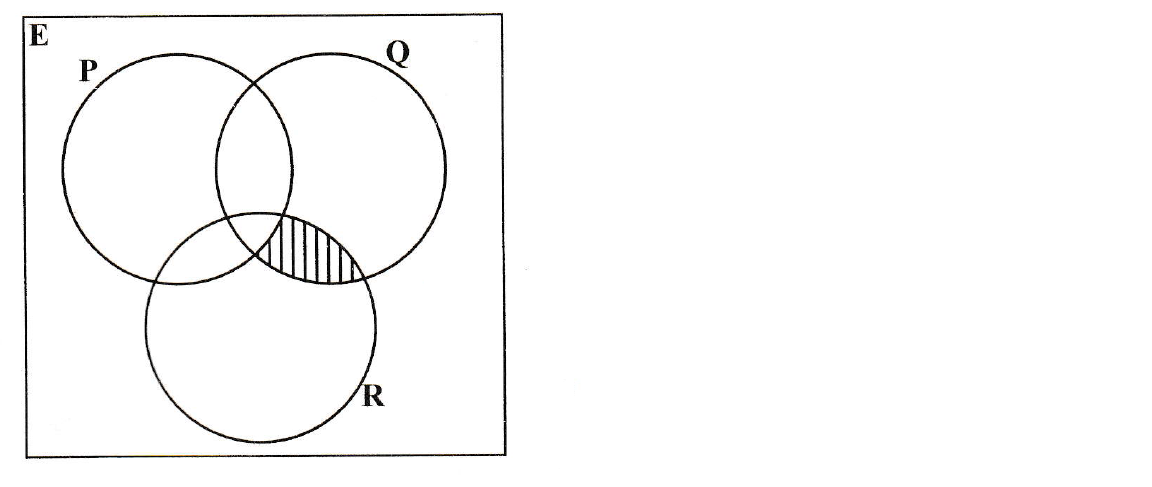The table below shows the relationship between two variables x and y. It is given that y varies inversely as the square root of x, where x is positive.

$\begin{array}{|llll|}\hline y& 2& 8& \frac{8}{9}\\ x& 16& 1& a\\ \hline\end{array}$

a) Write an expression for y in terms of x and the constant of variation k.

b) Find the value of

(i) k,

(ii) a.

1. a) $y=\frac{k}{\sqrt{x}}$ b) (i) k = 8 (ii) a = 81

Solve the following simultaneous equations

a)

2x + 3y = 5
x -4y = 8

b)

2x + 5y = 16
3x - 2y = 5

c)

4x +3y = 12
2x - 5y = 12.5

a) x = 4, y = -1 b) x = 3 , y = 2 c) x = 3.75, y = -1

1. The function g and f are defined as g:x ---> $\frac{\mathrm{x - 1}}{2}$ and f:x ---> $3x - 5$

a) ${g}^{-1}\left(x\right)$ b) x, if f(x) = g(x) c) ${g}^{-1}f\left(x\right)$

2. Given that f(x) = 8x and g(x) = $\frac{\mathrm{3x - 2}}{4}$

a) ${f}^{-1}\left(x\right)$

b) an expression for fg(x)

c) the value of x for which fg(x) = 20

1. a) ${g}^{-1}\left(x\right)=2x + 1$

b) x = $1\frac{4}{5}$

c) $3 \left(2x - 3\right)$

2. a) ${f}^{-1}\left(x\right)$ = $\frac{x}{8}$

b) fg(x) = 2(3x - 2)

c) x = 4

solve the following matrices

a)

M = $\left[\begin{array}{cc}2& 3\\ -1& 5\end{array}\right]$ and N = $\left[\begin{array}{c}2\\ 3\end{array}\right]$

(i) Determinant of M

(ii) Inverse of M

(iii) Value of MN

b)

Given that A = $\left[\begin{array}{cc}2& 1\\ -3& 4\end{array}\right]$ and B = $\left[\begin{array}{cc}-3& 0\\ 1& 2\end{array}\right]$

(i) 2B

(ii) A - 2B

c)

L = $\left[\begin{array}{cc}1& x\\ -1& 2\end{array}\right]$

(i) Find the value of x given that the determinant of L = 5

a)

(i) Determinant M = 13

(ii) Inverse of M = $\frac{1}{13}$ $\left[\begin{array}{cc}5& -3\\ 1& 2\end{array}\right]$

(iii) MN = $\left[\begin{array}{c}13\\ 13\end{array}\right]$

b)

(i) 2B = $\left[\begin{array}{cc}-6& 0\\ 2& 4\end{array}\right]$

(ii) A - 2B = $\left[\begin{array}{cc}8& 1\\ -5& 0\end{array}\right]$

c)

(i) x = 11

Solve the following Inequatities

a) 9j - 4 < 12j - 10

b) -5 < 2p + 3 < 1

c) 3 - 4a > 11

d) 4(1 - 2p) $\ge$ 32

a) j > 2

b) -4 < p < -1

c) a < -2

d) p $\le$ -3.5

a)

Given that the equation of a straight line is 3y – 2x + 9 = 0.

b)

The points J and K are (4, -3) and (12, 7) respectively; find

(i) The Coordinates of the midpoint of JK,

(ii) The gradient of a straight line passing through JK

(iii) The length of line JK

(iv) The equation of the straight line JK

a) (i) M = $\frac{2}{3}$

b)

(i) Midpoint = (8, 2)

(ii) gradient of JK = $\frac{5}{4}$

(iii) length or distance JK = 12.8

(iv) Equation: 4y = 5x -32

(a) Solve the equation ${\mathrm{2x}}^{2}$ = 6x + 3, given your answers correct to 2 decimal places

(b) Use the quadratic equation formula to find the equation ${x}^{2}-6x-27=0$

(a) x = 3.44 or x = -0.44

(b) x = 9 or x = -3

a) An equilateral triangle has sides of length 6cm. Find the height of the triangle. In an equilateral triangle, the lengths of all sides are equal. In the diagram on the right, the height is AD. BD is 3cm because of half of BC.

Find h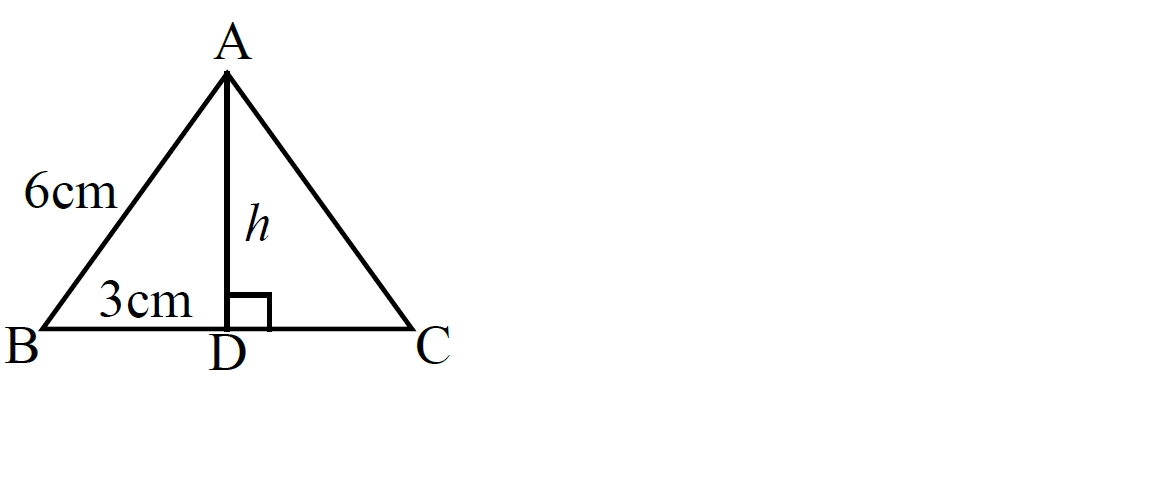b) Find the length of AC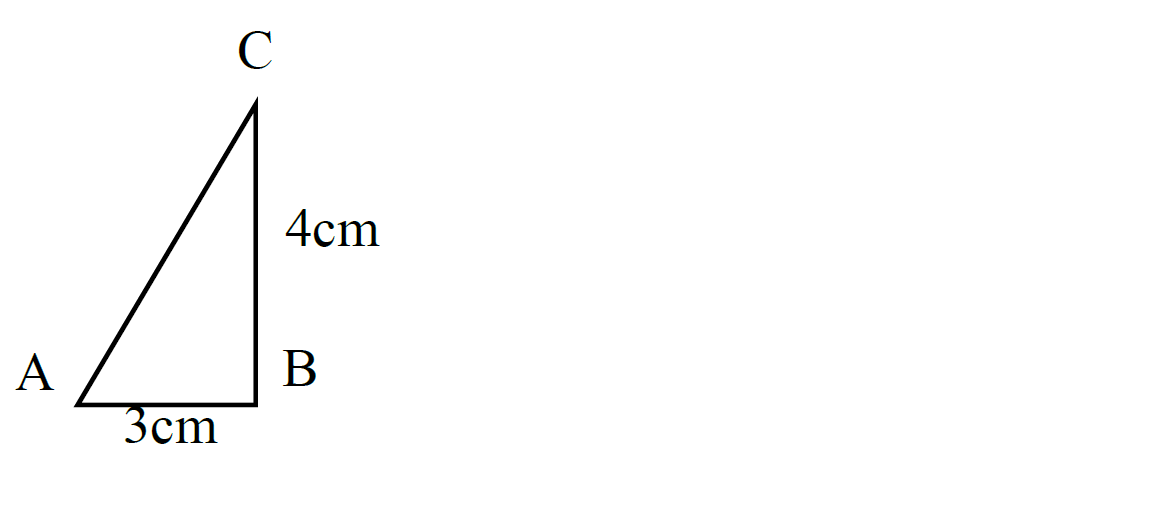a) h = 5.20cm b) 5cm

a) In the diagram below, ABC is at a tangent to the circle centre O and BE is the diameter. < ABF = 68 and < DFE = 35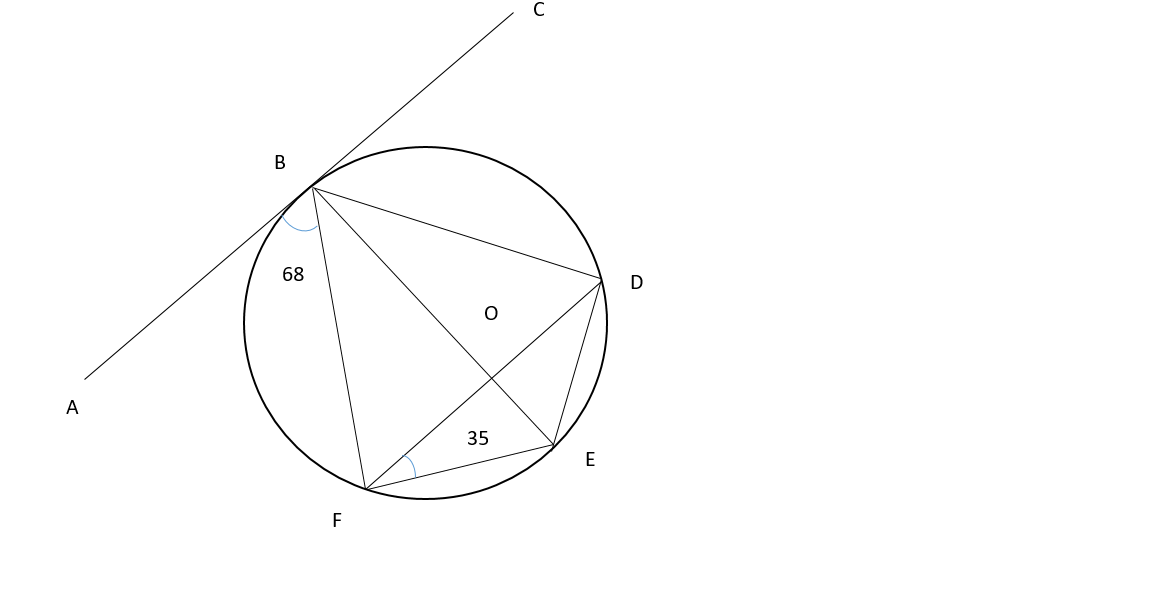(i) Explain why < EBC = 90

(ii) < BFD

(iii) < DBC

(iv) < FED

(v) < FDB

(i) The radius is perpendicular to the tangent of a circle at the point of contact.

(ii) < BFD = ${\mathrm{55}}^{o}$

(iii) < DBC = ${\mathrm{55}}^{o}$

(iv) < FED = ${\mathrm{123}}^{o}$

(v) < FDB = ${\mathrm{68}}^{o}$

In the diagram OPQR is a parallelogram, M is the mid-point of PQ and N is the mid-point of QR. OP = 2a, and OR = 2b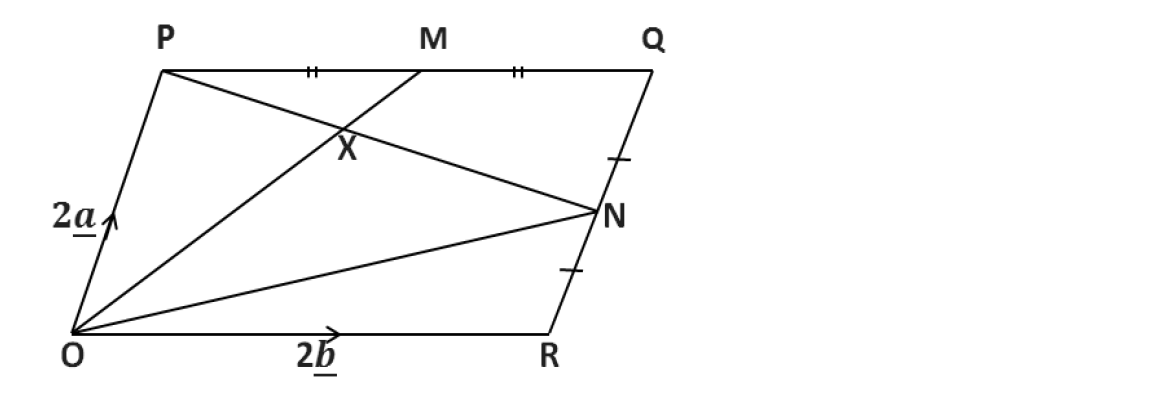Express in terms of a and/ or b the vectors

(a) PM

(b) OM

(c) ON

(d) PN

(a) PM = b (b) OM = 2a + b (c)ON = 2b + a (d) PN = 2b - a

X, Y and Z are points on the surface of the Earth as shown in the diagram below. X is on the parallel of latitude 55⁰N, Z and Y are on the same parallel of latitude 65⁰S, X and Y are on the same longitude 30⁰E and Z is on longitude 45⁰W. [𝜋 = 3.142,𝑅 = 6370 𝑘𝑚].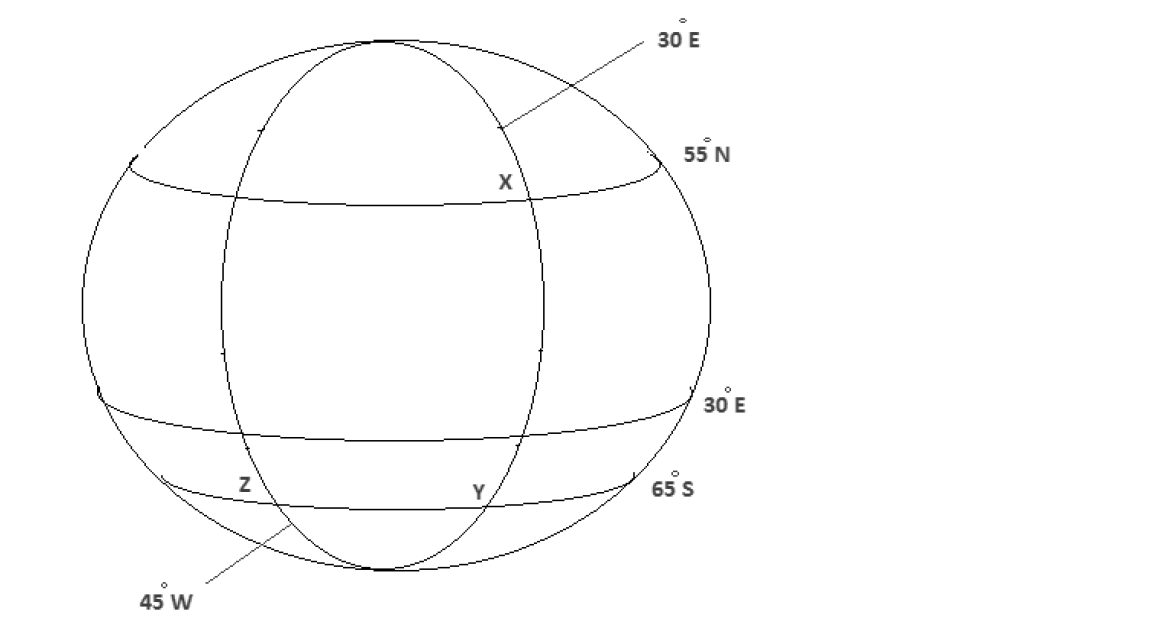(a) Find the difference in latitude between points X, Y

(b) Calculate the distance XY along the same longitude, giving your answer to the nearest kilometre.

(c) If the local time at Z is 06: 00 hours, what is the time at Y.

(d) Find the distance ZY in kilometres, giving your answer to the nearest kilometre.

(a) 120⁰ (b) 13343km (c) 11 : 00 hours (d) 3524km